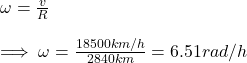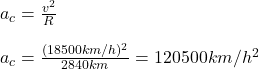## What are the magnitudes of (a) the angular velocity, (b) the radial acceleration, and (c) the tangential acceleration of a spaceship taking

Question

What are the magnitudes of (a) the angular velocity, (b) the radial acceleration, and (c) the tangential acceleration of a spaceship taking a circular turn of radius 2840 km at a speed of 18500 km/h

in progress 0
6 months 2021-08-26T05:01:44+00:00 1 Answers 3 views 0

## Answers ( )

(b) 120500km/h²

(c) Zero

Explanation:

The angular velocity of an object in circular motion is given by:So the angular velocity has magnitude 6.51rad/h, roughly 1.03rev/h (a).

The radial (centripetal) acceleration is given by:In words, the radial acceleration is roughly equal to 120500km/h² (b).

Since the spaceship is describing a circular motion with constant speed, the magnitude of the acceleration parallel to its velocity is zero (because its speed isn’t changing). So, the magnitude of the tangential acceleration is zero (c).